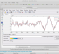## A question regarding MATLAB's 'invfreqz()' commandStarted by 3 years ago6 replieslatest reply 3 years ago202 views
Hi. When I execute the following MATLAB code:

b = [1 0 0 0 -1]
a = [1, -1]
[h, w] = freqz(b, a, 64);
b_Order = length(b) -1; a_Order = length(a) -1;
[bb, aa] = invfreqz(h, w, b_Order, a_Order)

I expect to produce

bb =

1  0  0  0  -1
aa =

1   -1

But instead of the above 'bb' and 'aa' sequences my code produces:

bb =

NaN   NaN   NaN   NaN   NaN
aa =

1   NaN

Can someone here tell me what's going on here? Thanks.
[ - ]if you change a=[1,-0.9] everything works as you expect. With your example the array h has NaN in the first position  I guess that is the problem.

[ - ]Hi anamariatome.
Thanks for pointing out that h(1) = NaN condition.
(I hadn't noticed that.) To correct for that h(1) = NaN condition, I replaced my '[h,w] = freqz(b, a, 1024);' command with:

[h,w] = freqz(b, a, 1024);
h(1) = sum(ImpResp);

But with that change in my code I still obtained wildly incorrect values for variables 'bb' and 'aa'.

Following your suggestion, going back to my original code, when I redefined the denominator 'a' coefficients as:

a = [1, -.9999]

to move the pole very slightly inside the unit circle I obtained correct 'bb' and 'aa' results! So it's not the h(1) = NaN condition causing me problems, it's the pole at z = 1 causing a divide by zero condition inside MATLAB's invfreqz() command. Moving that pole to very slightly inside the unit circle is the thing to do.

I think this all means that MATLAB's invfreqz() command has a "bug."

Thanks again.

[ - ]Hi Rick,

I would think the dc gain of your filter is to blame.

iir dc gain = sum(b)/sum(a) = 0/0 = NAN because of divide by zero... if I am right...

[ - ]Hi Mr Lyons,

First value of h is NaN as I have checked. Maybe that is the reason?

Edit: (As noted by  also, that is the problem indeed, pole at z=1(f=0) causing NaN value) I tried with other a,b values

[ - ]Hi omersayli. Please call me "Rick." My friends do.

Thanks for your thoughts, and you are correct. Check out my Oct. 22 Reply to anamariatome.

[ - ]You are right Rick ,

b = [1 0 0 0 -1]

a = [1 -0.9]

freqz_points = 512

[h, w] = freqz(b, a, freqz_points);

[imp,t] = impz(b,a);

h(1) = sum(imp)

b_Order = length(b)-1; a_Order = length(a)-1;

[bb, aa] = invfreqz(h, w, b_Order, a_Order)

trying this, it works (I substituted h(1) with impz result as you suggested also). When trying   a = [1 -1], you get different results for different "freqz_points" parameter! That is really weird

EDIT: Interestingly, When making h(1) = 0 , the 'invfreqz' function works quite good, at least for the a, b arrays I have tried.